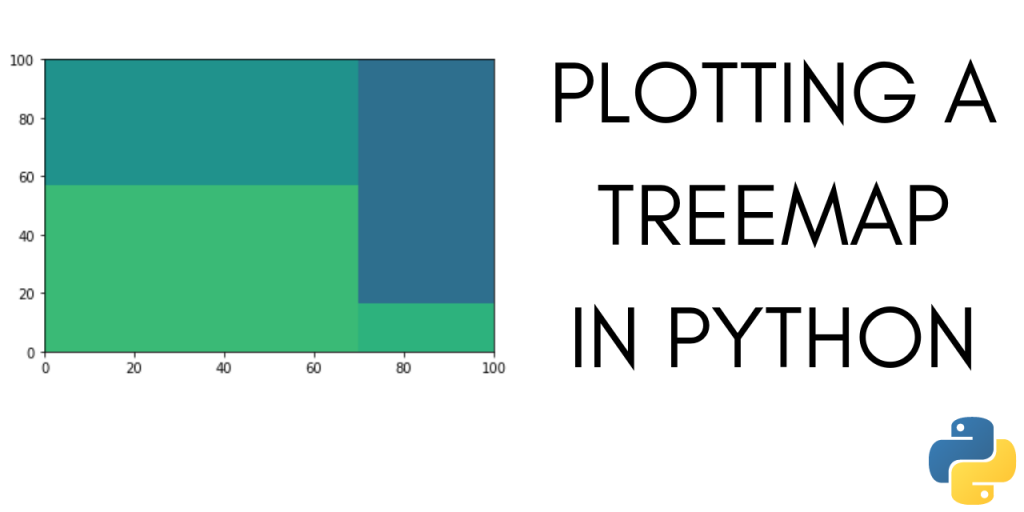# How to Plot a Treemap in Python?A treemap in Python is a visualization of data that splits a rectangle into sub-parts. The size of each subpart is in proportion to the data it represents. It is somewhat like a pie-chart. Although, treemaps can represent much-more complex data as compared to a pie-chart.

It can help you visualize how single values compose a whole. Treemap charts also let you visualize hierarchical data using nested rectangles.

In this tutorial, we will learn how to plot treemaps in Python using the Squarify library in python.

Let’s start by installing Squarify.

```pip install squarify
```

## Using Squarify to Plot a Treemap in Python

Once we have installed Squarify, we can start by importing it into our notebook. Let’s also import matplotlib.

```import matplotlib.pyplot as plt
import squarify
```

### 1. Plotting a basic treemap

To plot a very basic treemap, we just need the values for each rectangle. After plotting the treemap, the rectangles would be in proportion to these values.

```import matplotlib.pyplot as plt
import squarify
sizes = [40, 30, 5, 25]
squarify.plot(sizes)
plt.show()
```

You can add labels to the treemap in Python, using the following lines of code :

```import matplotlib.pyplot as plt
import squarify
sizes=[40, 30, 5, 25]
label=["A", "B", "C", "D"]
squarify.plot(sizes=sizes, label=label, alpha=0.6 )
plt.show()
```

If you run the same piece of code again, you will get the following output :

You can see that the color scheme of our treemap is different each time we run it. The colors for rectangles are picked randomly. Treemap also gives you the option to mention the colors along with sizes and labels. We will learn how to change the colors of a treemap next.

### 3. Change the colors in your treemap

To change the colors in your treemap in Python, make a list with the colors you want the treemap to have. Then pass that list to squarify.plot method.

```import matplotlib.pyplot as plt
import squarify
sizes=[40, 30, 5, 25]
label=["A", "B", "C", "D"]
color=['red','blue','green','grey']
squarify.plot(sizes=sizes, label=label, color=color, alpha=0.6 )
plt.show()
```

### 4. Turn-off the plot axis

To plot the treemap without the plot-axis, use:

```plt.axis('off')
```

This line of code will turn off the plot axis. The complete code is as follows:

```import matplotlib.pyplot as plt
import squarify
sizes=[40, 30, 5, 25]
label=["A", "B", "C", "D"]
color=['red','blue','green','grey']
squarify.plot(sizes=sizes, label=label, color=color, alpha=0.6 )
plt.axis('off')
plt.show()
```

## Plot treemap for a Dataset

In this part of the tutorial, we will learn how to plot a treemap for a dataset. We are going to use the titanic dataset. Let’s start by importing the dataset. To simplify the process of importing the dataset we are going to use the seaborn library.

### 1. Importing the dataset

To import the titanic dataset from the seaborn library into your Python notebook, use:

```import seaborn as sns
```

The dataset contains information about the passengers of Titanic.

We want to plot a treemap for the people who survived according to the class they were travelling in.

The data in its original format is not ready for plotting a treemap. We will carry out some manipulations and try to extract data that we can use to plot a treemap.

To get the survivors for each class we are going to use group by method on our data.

### 2. Preparing the Data for Plotting

You can use the groupby function on the dataset as shown below :

```n = titanic.groupby('class')[['survived']].sum()
```

This gives us the sum of total survivors grouped according to the class.

Now we need to extract the data and labels as lists from this.

```a = titanic.groupby('class')[['survived']].sum().index.get_level_values(0).tolist()
print(a)
```

Output :

```['First', 'Second', 'Third']
```

This gives us the labels in the form of a list. To get the values corresponding to these labels, use :

```d = titanic.groupby('class')[['survived']].sum().reset_index().survived.values.tolist()
print(d)
```

Output :

```[136, 87, 119]
```

Now we have the labels and data as lists. We can use these to plot a treemap.

### 3. Plot the treemap

To plot the treemap, use the following line of code :

```squarify.plot(sizes=d, label=a, alpha=.8)
plt.axis('off')
plt.show()
```

Visualizing the treemap, we can get a rough idea about the number of survivors in the first, second, and third class. Just by looking at the treemap, we can confidently say that the second class has the least number of survivors.

### Complete code to plot a treemap in Python

The complete code from this section is given below :

```import seaborn as sns
import squarify
import matplotlib.pyplot as plt

a = titanic.groupby('class')[['survived']].sum().index.get_level_values(0).tolist()

d = titanic.groupby('class')[['survived']].sum().reset_index().survived.values.tolist()

squarify.plot(sizes=d,label=a, alpha=.8 )
plt.axis('off')
plt.show()

```

## Conclusion

In this tutorial, we learned how to plot a treemap in python using Squarify. Hope you had fun learning with us.